# Limit and Colimit

I often forget the conceptual background of these well known universal constructions. This bothers me especially when I need to give a new mathematical instrument the conceptual meaning as the extended or restricted version of limit or colimit. Here I write of limit/colimit that include definitions and some concrete examples of instantiating the concept, in order for reminding me of least rigoristic idea that activate the lost memory.

Definition 1. Let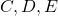are categories and functors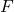and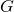are given as: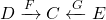. The comma category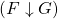is a category composed of a triple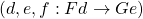as an object and the morphism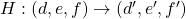is a pair of morphisms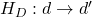and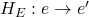(we write these only as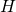) that commutes the following diagram:Note that this definition generalizes the case when a functor is an object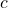of the category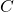, sincecan be regarded as the functor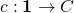. In this sense, when both the functors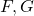are objects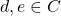, then the corresponding comma category is exactly the hom-set written as: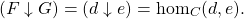Definition 2. Let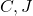be categories (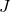is called index category, usually taken to be small or finite). The diagonal functor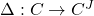is the functor that maps for each object the constant functor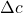, and for each morphism, the morphism function that assigns each index constantly to the given morphism as in the diagram:Definition 3. Let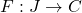be a functor. If exists, the initial object of comma category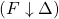, regarding the functors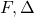as to fit in the sequence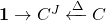, is called the colimit (of), denoted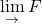.

Definition 4. Letbe a functor. If exists, the terminal object of comma category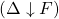, regarding the functors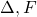as to fit in the sequence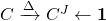, is called the limit (of), denoted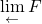.

Example 1. Coproduct is the colimit of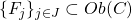, regarding the corresponding functoras indexed objects. We usually denote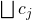for the coproduct of given indexed objects. In the following diagram, the colimit as the universal arrow is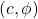.Example 2. Letbe a category of two objects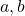and two morphisms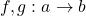. Then an object of the category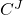is exactly a pair of morphisms, also denotein. We call the colimit of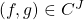the coequalizer (of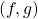). An object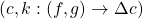of comma category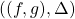can be represented by a commutative diagram:Hence the coequalizer ofis the pair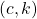such that for any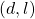, there is unique morphism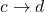inthat commutes the following diagram:Example 2.1. Whenis the category of abelian group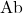or the category of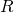-module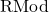for a certain ring, and suppose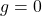, then the coequalizer ofis the quotient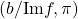.

Example 3. Letdenotes an index category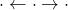, then an object ofis again of the same shape, namely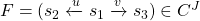. Any element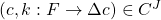suffices the commutative diagram:hence the colimit of, called pushout of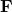, is a pair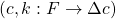that for any pair, there exists unique morphismsuch that the following diagram commutes: